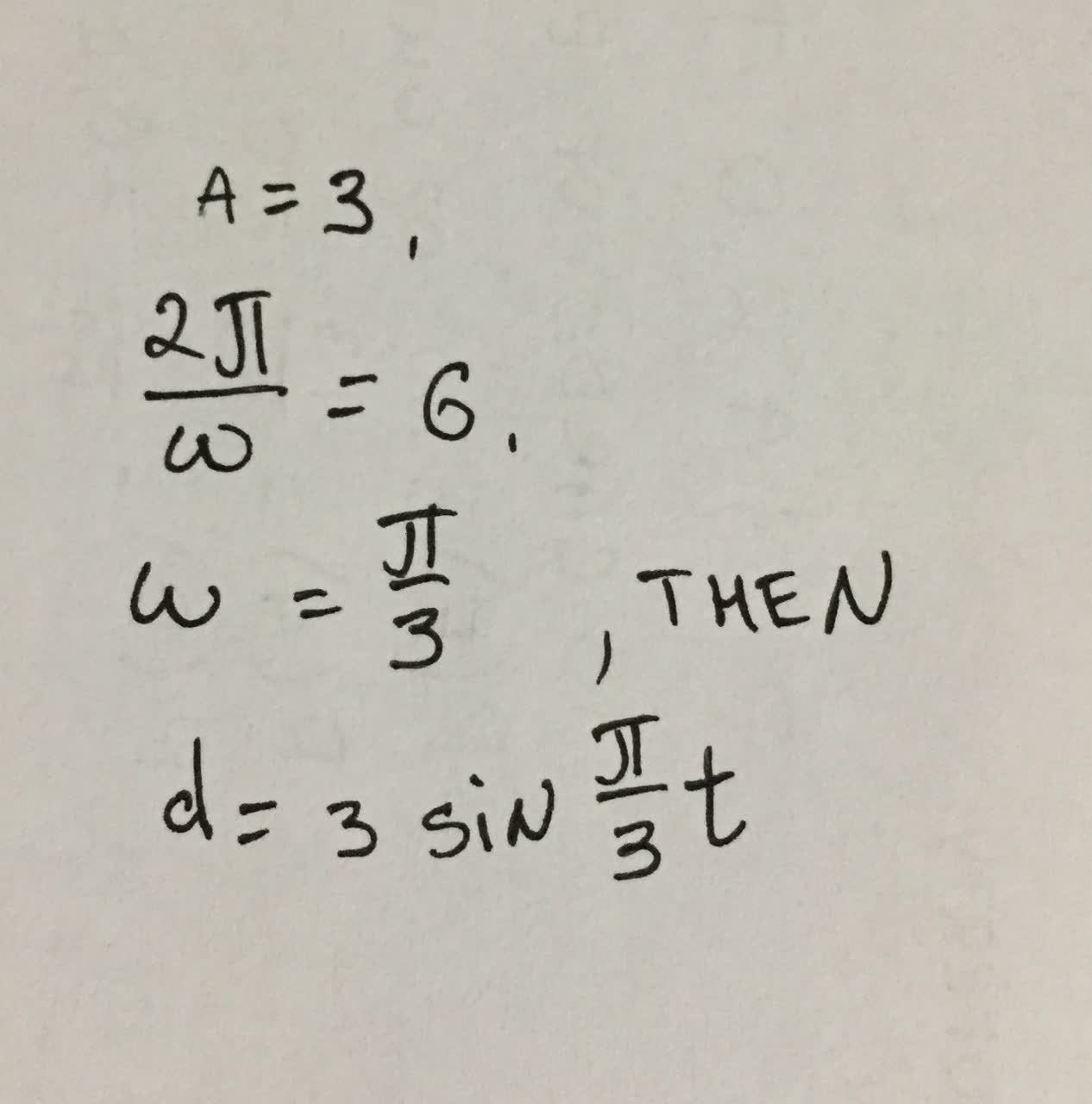# Please, help to find a model for simple harmonic motion satisfying the specified conditions. Displacement (t = 0) 0, Amplitude 3 metersfoass77W 2021-09-18 Answered
Please, help to find a model for simple harmonic motion satisfying the specified conditions. Displacement (t = 0) 0, Amplitude 3 meters, Period 6 seconds.

• Questions are typically answered in as fast as 30 minutes

### Plainmath recommends

• Get a detailed answer even on the hardest topics.
• Ask an expert for a step-by-step guidance to learn to do it yourself.yagombyeR

$$\displaystyle{a}={3}$$
$$\displaystyle{2}\frac{\pi}{\omega}={6}$$
$$\displaystyle\omega=\frac{\pi}{{3}}$$
$$\displaystyle{d}={}\frac{{3\sin{\pi}}}{{3}}\times{t}$$

###### Have a similar question?content_user
Here is right answer